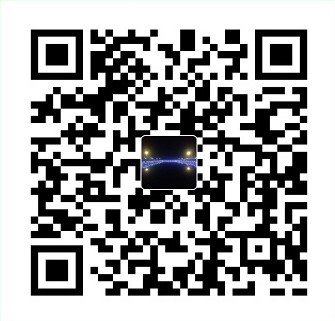• 端到端的检测+识别框架
• 在处理不规则的文本形状时，优于之前的方法
• 除了文本行检测外，能进行字符分割

## 介绍

1. 提出了一种端到端的文本检测加识别模型，具有简单高效的特点
2. 该方法可以检测和识别各种形状的文本，包括水平文本、定向文本和弯曲文本
3. 与之前的方法相比，该方法通过语义分割来实现精确的文本检测和识别
4. 在各种基准的文本检测和定位上都达到了state-of-the-art的效果

## 方法

### 框架

该架构由以下4部分组成

• backbone：ResNet50；FPN网络（ top-down 结构）
• 生成文本建议：RPN
• 边界框回归：Fast R-CNN

每部分的细节如下：

• Backbone:自然场景的文本大小不一，为了提取更高层的语义特征。采用了ResNet-50网络，并用对小目标有较好效果的FPN网络提取特征
• RPN:RPN网络为Fast R-CNN分支以及mask分支生成文本建议。anchor设置5种尺寸$\{32^2,64^2,128^2,256^2,512^2\}$，FPN网络中有5个层级$\{P_2,P_3,P_4,P_5\}$，3种比例$\{0.5,1,2\}$。区域特征映射方式采用ROI Align。
• Fast R-CNN:包括分类和回归任务，主要作用是为了后续的检测提供更精确的检测框。
• 全局文本实例分割
• 背景分割
• 10个数字，26个字母

### 标签的生成

为了满足训练的要求，ground truth要包含$P=P\{p_1,p_2…p_m\}$以及$C=\{c_1=(cc_1,cl_1),c_2=(cc_2,cl_2),…,c_n=(cc_n,cl_n)\}$。其中$p_i$表示一个文本定位的多边形。$cc_j$和$cl_j$分别是字符的类别和定位。值得一提的是并不要求所有样本都需要有标记$C$。

其中$(B_{x0},B_{y0})$是所有多边形和字符原始的顶点，$(B_x,B_y)$是所有多变形和字符更新后的顶点，$(r_x,r_y)$是rpoposal产生的顶点。

### 损失函数

mask分支有两个任务，因此$L_{mask}$的计算如下：

其中$L_{global}$为Cross entropy损失，而$L_{char}$为Softmax损失。其中作者设置的超参数$α_1,α_2,β$都为1。
$L_{global}$的计算公式如下：

$L_{char}$的计算公式如下：

其中T为类别，X为预测的输出，Y为gt，W为权重。W的主要作用是为了样本均衡。不同类别的W计算公式如下：

### 推理(测试)

1. 输入测试图片，获得Fast R-CNN的输出，并经过NMS;
3. 通过计算全局映射的map区域获得预测的多边形，通过pixel voting算法生成字符映射序列

pixel voting算法的细节如下：

1. 二值化背景图，阈值为192
2. 根据二值化中的联通与划分所有字符区域

## 实验

作者总共使用了4个数据集，除了SynthText用来预训练以外，其余的三个数据集ICDAR 2013，ICDAR2015，Total_Text均做了测试实验。微信支付宝